## WitCH 65: Repeat Offender

The following is our second WitCH from the 2021 NHT Methods Exam 2.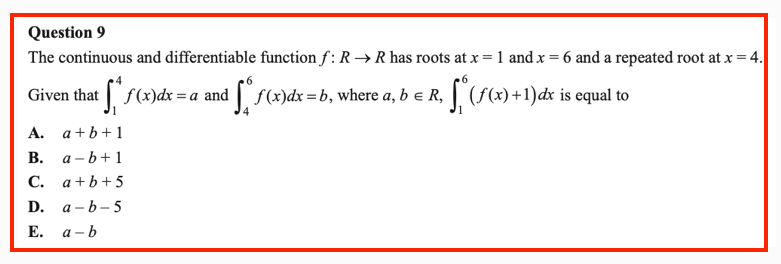## WitCH 58: Differently Abled

Like the previous post, this one comes from Maths Quest Mathematical Methods 11, and is most definitely a WitCH. It can also been seen as a “contrast and compare” with WitCH 15.

Subsection 13.2.5, below, is on “differentiability”. The earlier part of chapter 13 gives a potted, and not error-free, introduction to limits and continuity, and Chapter 12 covers the “first principles” (limit) computation of polynomial derivatives. We’ve included the relevant “worked example”, and the relevant exercises and answers.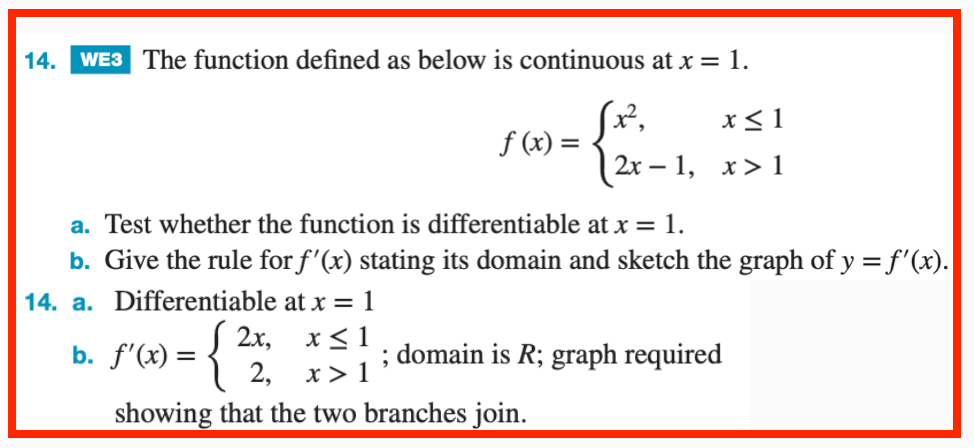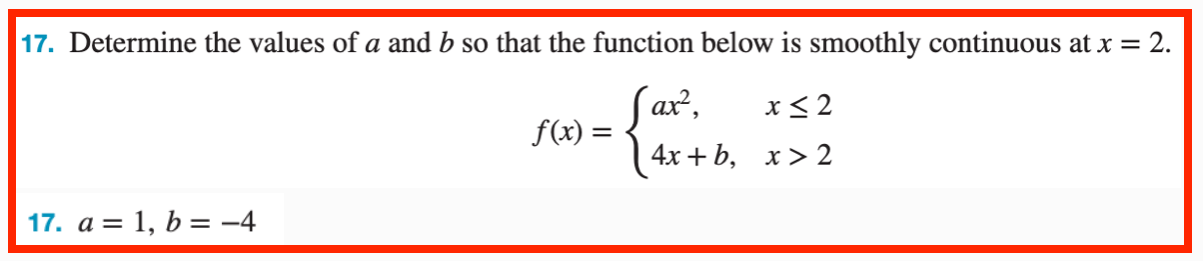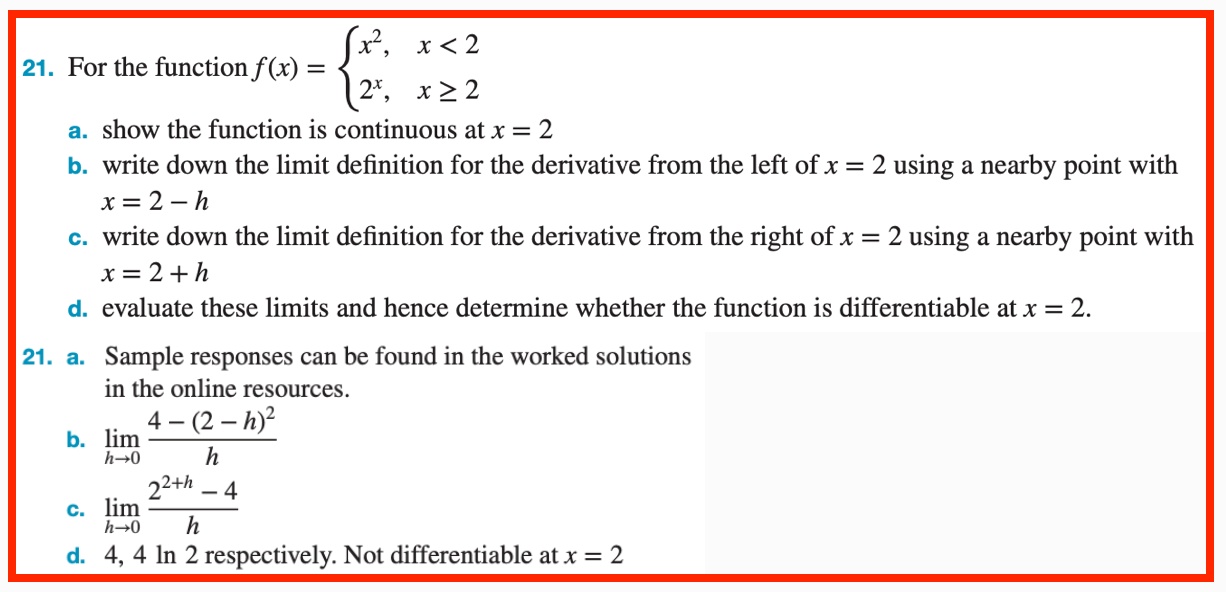## WitCH 15: Principled Objection

OK, playtime is over. This one, like the still unresolved WitCH 8, will take some work. It comes from Cambridge’s Mathematical Methods 3 & 4 (2019). It is the introduction to “When is a function differentiable?”, the final section of the chapter “Differentiation”.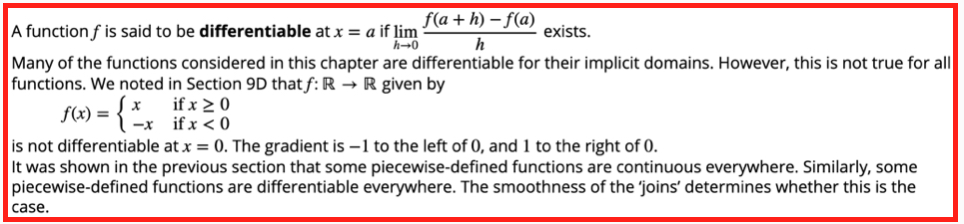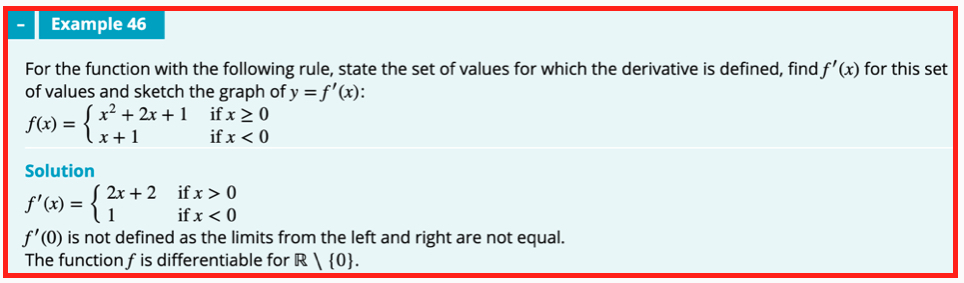## Update (12/08/19)

We wrote about this nonsense seven long years ago, and we’ll presumably be writing about it seven years from now. Nonetheless, here we go.

The first thing to say is that the text is wrong. To the extent that there is a discernible method, that method is fundamentally invalid. Indeed, this is just about the first nonsense whacked out of first year uni students.

The second thing to say is that the text is worse than wrong. The discussion is clouded in gratuitous mystery, with the long-delayed discussion of “differentiability” presented as some deep concept, rather than simply as a grammatical form. If a function has a derivative then it is differentiable. That’s it.

Now to the details.

The text’s “first principles” definition of differentiability is correct and then, immediately, things go off the rails. Why is the function f(x) = |x| (which is written in idiotic Methods style) not differentiable at 0? The wording is muddy, but example 46 makes clear the argument: f’(x) = -1 for x < 0 and f’(x) = 1 for x > 0, and these derivatives don’t match. This argument is unjustified, fundamentally distinct from first principles, and it can easily lead to error. (Amusingly, the text’s earlier, “informal” discussion of f(x) = |x| is exactly what is required.)

The limit definition of the derivative f’(a) requires looking precisely at a, at the gradient [f(a+h) – f(a)]/h as h → 0. Instead, the text, with varying degrees of explicitness and correctness, considers the limit of f’(x) near a, as x → a. This second limit is fundamentally, conceptually different and it is not guaranteed to be equal.

The standard example to illustrate the issue is the function f(x) = x2sin(1/x) (for x≠ 0 and with f(0) = 0). It is easy to to check that f’(x) oscillates wildly near 0, and thus f’(x) has no limit as x → 0. Nonetheless, a first principles argument shows that f’(0) = 0.

It is true that if a function f is continuous at a, and if f’(x) has a limit L as x → a, then also f’(a) = L. With some work, this non-obvious truth (requiring the mean value theorem) can be used to clarify and to repair the text’s argument. But this does not negate the conceptual distinction between the required first principles limit and the text’s invalid replacement.

Now, to the examples.

Example 45 is just wrong, even on the text’s own ridiculous terms. If a function has a nice polynomial definition for x ≥ 0, it does not follow that one gets f’(0) for free. One cannot possibly know whether f’(x) exists without considering x on both sides of 0. As such, the “In particular” of example 46 is complete nonsense. Further, there is the sotto voce claim but no argument that (and no illustrative graph indicating) the function f is continuous; this is required for any argument along the text’s lines.

Example 46 is wrong in the fundamental wrong-limit manner described above. it is also unexplained why the magical method to obtain f’(0) in example 45 does not also work for example 46.

Example 47 has a “solution” that is wrong, once again for the wrong-limit reason, but an “explanation” that is correct. As discussed with Damo in the comments, this “vertical tangent” example would probably be better placed in a later section, but it is the best of a very bad lot.

And that’s it. We’ll be back in another seven years or so.